International
Tables for
Crystallography
Volume D
Physical properties of crystals
Edited by A. Authier

International Tables for Crystallography (2006). Vol. D, ch. 2.2, pp. 301-303

## Section 2.2.11. Band-theory methods

K. Schwarza*

aInstitut für Materialchemie, Technische Universität Wien, Getreidemarkt 9/165-TC, A-1060 Vienna, Austria
Correspondence e-mail: kschwarz@theochem.tuwein.ac.at

### 2.2.11. Band-theory methods

| top | pdf |

There are several methods for calculating the electronic structure of solids. They have advantages and disadvantages, different accuracies and computational requirements (speed or memory), and are based on different approximations. Some of these aspects have been discussed in Section 2.2.9.This is a rapidly changing field and thus only the basic concepts of a few approaches in current use are outlined below.

#### 2.2.11.1. LCAO (linear combination of atomic orbitals)

| top | pdf |

For the description of crystalline wavefunctions (Bloch functions), one often starts with a simple concept of placing atomic orbitals (AOs) at each site in a crystal denoted by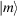, from which one forms Bloch sums in order to have proper translational symmetry: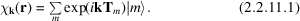Then Bloch functions can be constructed by taking a linear combination of such Bloch sums, where the linear-combination coefficients are determined by the variational principle in which a secular equation must be solved. The LCAO can be used in combination with both the Hartree–Fock method and DFT.

#### 2.2.11.2. TB (tight binding)

| top | pdf |

A simple version of the LCAO is found by parameterizing the matrix elements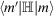and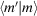in a way similar to the Hückel molecular orbital (HMO) method, where the only non-vanishing matrix elements are the on-site integrals and the nearest-neighbour interactions (hopping integrals). For a particular class of solids the parameters can be adjusted to fit experimental values. With these parameters, the electronic structures of rather complicated solids can be described and yield quite satisfactory results, but only for the class of materials for which such a parametrization is available. Chemical bonding and symmetry aspects can be well described with such schemes, as Hoffmann has illustrated in many applications (Hoffmann, 1988). In more complicated situations, however, such a simple scheme fails.

#### 2.2.11.3. The pseudo-potential schemes

| top | pdf |

In many respects, core electrons are unimportant for determining the stability, structure and low-energy response properties of crystals. It is a well established practice to modify the one-electron part of the Hamiltonian by replacing the bare nuclear attraction with a pseudo-potential (PP) operator, which allows us to restrict our calculation to the valence electrons. The PP operator must reproduce screened nuclear attractions, but must also account for the Pauli exclusion principle, which requires that valence orbitals are orthogonal to core ones. The PPs are not uniquely defined and thus one seeks to satisfy the following characteristics as well as possible:

 (1) PP eigenvalues should coincide with the true (all-electron) ones; (2) PP orbitals should resemble as closely as possible the all-electron orbitals in an external region as well as being smooth and nodeless in the core region; (3) PP orbitals should be properly normalized; (4) the functional form of the PP should allow the simplification of their use in computations; (5) the PP should be transferable (independent of the system); and (6) relativistic effects should be taken into account (especially for heavy elements); this concerns mainly the indirect relativistic effects (e.g. core contraction, Darwin s-shift), but not the spin–orbit coupling.

There are many versions of the PP method (norm-conserving, ultrasoft etc.) and the actual accuracy of a calculation is governed by which is used. For standard applications, PP techniques can be quite successful in solid-state calculations. However, there are cases that require higher accuracy, e.g. when core electrons are involved, as in high-pressure studies or electric field gradient calculations (see Section 2.2.15), where the polarization of the charge density close to the nucleus is crucial for describing the physical effects properly.

#### 2.2.11.4. APW (augmented plane wave) and LAPW methods

| top | pdf |

The partition of space (i.e. the unit cell) between (non-overlapping) atomic spheres and an interstitial region (see Fig. 2.2.12.1) is used in several schemes, one of which is the augmented plane wave (APW) method, originally proposed by Slater (Slater, 1937) and described by Loucks (1967), and its linearized version (the LAPW method), which is chosen as the one representative method that is described in detail in Section 2.2.12.

The basis set is constructed using the muffin-tin approximation (MTA) for the potential [see the discussion below in connection with (2.2.12.5)]. In the interstitial region the wavefunction is well described by plane waves, but inside the spheres atomic-like functions are used which are matched continuously (at the sphere boundary) to each plane wave.

#### 2.2.11.5. KKR (Korringa–Kohn–Rostocker) method

| top | pdf |

In the KKR scheme (Korringa, 1947; Kohn & Rostocker, 1954), the solution of the KS equations (2.2.10.3)uses a Green-function technique and solves a Lippman–Schwinger integral equation. The basic concepts come from a multiple scattering approach which is conceptually different but mathematically equivalent to the APW method. The building blocks are spherical waves which are products of spherical harmonics and spherical Hankel, Bessel and Neumann functions. Like plane waves, they solve the KS equations for a constant potential. Augmenting the spherical waves with numerical solutions inside the atomic spheres as in the APW method yields the KKR basis set. Compared with methods based on plane waves, spherical waves require fewer basis functions and thus smaller secular equations.

The radial functions in the APW and KKR methods are energy-dependent and so are the corresponding basis functions. This leads to a nonlinear eigenvalue problem that is computationally demanding. Andersen (1975) modelled the weak energy dependence by a Taylor expansion where only the first term is kept and thereby arrived at the so-called linear methods LMTO and LAPW.

#### 2.2.11.6. LMTO (linear combination of muffin-tin orbitals) method

| top | pdf |

The LMTO method (Andersen, 1975; Skriver, 1984) is the linearized counterpart to the KKR method, in the same way as the LAPW method is the linearized counterpart to the APW method. This widely used method originally adopted the atomic sphere approximation (ASA) with overlapping atomic spheres in which the potential was assumed to be spherically symmetric. Although the ASA simplified the computation so that systems with many atoms could be studied, the accuracy was not high enough for application to certain questions in solid-state physics.

Following the ideas of Andersen, the augmented spherical wave (ASW) method was developed by Williams et al. (1979). The ASW method is quite similar to the LMTO scheme.

It should be noted that the MTA and the ASA are not really a restriction on the method. In particular, when employing the MTA only for the construction of the basis functions but including a generally shaped potential in the construction of the matrix elements, one arrives at a scheme of very high accuracy which allows, for instance, the evaluation of elastic properties. Methods using the unrestricted potential together with basis functions developed from the muffin-tin potential are called full-potential methods. Now for almost every method based on the MTA (or ASA) there exists a counterpart employing the full potential.

#### 2.2.11.7. CP (Car–Parrinello) method

| top | pdf |

Conventional quantum-mechanical calculations are done using the Born–Oppenheimer approximation, in which one assumes (in most cases to a very good approximation) that the electrons are decoupled from the nuclear motion. Therefore the electronic structure is calculated for fixed atomic (nuclear) positions. Car & Parrinello (1985) suggested a new method in which they combined the motion of the nuclei (at finite temperature) with the electronic degrees of freedom. They started with a fictitious Lagrangian in which the wavefunctions follow a dynamics equation of motion. Therefore, the CP method combines the motion of the nuclei (following Newton's equation) with the electrons (described within DFT) into one formalism by solving equations of motion for both subsystems. This simplifies the computational effort and allows ab initio molecular dynamics calculations to be performed in which the forces acting on the atoms are calculated from the wavefunctions within DFT. The CP method has attracted much interest and is widely used, with a plane-wave basis, extended with pseudo-potentials and recently enhanced into an all-electron method using the projector augmented wave (PAW) method (Blöchl, 1994). Such CP schemes can also be used to find equilibrium structures and to explore the electronic structure.

#### 2.2.11.8. Order N schemes

| top | pdf |

The various techniques outlined so far have one thing in common, namely the scaling. In a system containing N atoms the computational effort scales as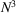, since one must determine a number of orbitals that is proportional to N which requires diagonalization of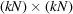matrices, where the prefactor k depends on the basis set and the method used. In recent years much work has been done to devise algorithms that vary linearly with N, at least for very large N (Ordejon et al., 1995). First results are already available and look promising. When such schemes become generally available, it will be possible to study very large systems with relatively little computational effort. This interesting development could drastically change the accessibility of electronic structure results for large systems.

### References

Andersen, O. K. (1975). Linear methods in band theory. Phys. Rev. B, 12, 3060–3083.
Blöchl, P. E. (1994). Projector augmented-wave method. Phys. Rev. B, 50, 17953–17979.
Car, R. & Parrinello, M. (1985). Unified approach for molecular dynamics and density-functional theory. Phys. Rev. Lett. 55, 2471–2474.
Hoffmann, R. (1988). Solids and surfaces: A chemist's view of bonding in extended structures. New York: VCH Publishers, Inc.
Kohn, W. & Rostocker, N. (1954). Solution of the Schrödinger equation in periodic lattice with an application to metallic lithium. Phys. Rev. 94, A1111–A1120.
Korringa, J. (1947). On the calculation of the energy of a Bloch wave in a metal. Physica, 13, 392–400.
Loucks, T. L. (1967). Augmented plane wave method. New York, Amsterdam: W. A. Benjamin, Inc.
Ordejon, P., Drabold, D. A., Martin, R. A. & Grumbach, M. P. (1995). Linear system-size scaling methods for electronic-structure calculations. Phys. Rev. B, 51, 1456–1476.
Skriver, H. L. (1984). The LMTO method. Springer series in solid-state sciences, Vol. 41. Berlin, Heidelberg, New York, Tokyo: Springer.
Slater, J. C. (1937). Wavefunctions in a periodic crystal. Phys. Rev. 51, 846–851.
Williams, A. R., Kübler, J. & Gelatt, C. D. Jr (1979). Cohesive properties of metallic compounds: Augmented-spherical-wave calculations. Phys. Rev. B, 19, 6094–6118.